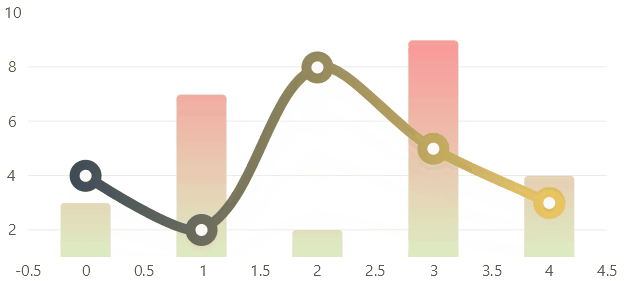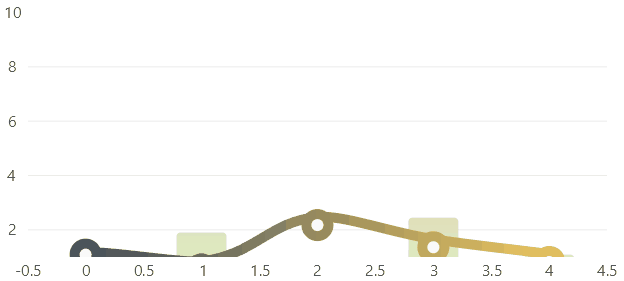Hover over the image to see the chart animation## View model

``````using LiveChartsCore;
using LiveChartsCore.SkiaSharpView;
using LiveChartsCore.SkiaSharpView.Painting;
using SkiaSharp;

public class ViewModel
{
public ViewModel()
{

var colors = new[]
{
new SKColor(45, 64, 89),
new SKColor(255, 212, 96)
// ...

// you can add as many colors as you require to build the gradient
// by default all the distance between each color is equal
// use the colorPos parameter in the constructor of the LinearGradientPaint class
// to specify the distance between each color
};

Series = new ISeries[]
{
new ColumnSeries<int>
{
Values = new []{ 3, 7, 2, 9, 4 },
Stroke = null,

// this is an easy way to set a linear gradient:
// Fill = new LinearGradientPaint(new SKColor(255, 140, 148), new SKColor(220, 237, 194))

// but you can customize the gradient
// the gradient will use the following colors array
new [] { new SKColor(255, 140, 148), new SKColor(220, 237, 194) },

// now with the following points we are specifying the orientation of the gradient
// by default the gradient is orientated horizontally
// defined by the points: (0, 0.5) and (1, 0.5)
// but for this sample we will use a vertical gradient:

// to build a vertical gradient we must specify 2 points that will draw a imaginary line
// the gradient will interpolate colors lineally as it moves following this imaginary line
// the coordinates of these points (X, Y) go from 0 to 1
// where 0 is the start of the axis and 1 the end. Then to build our vertical gradient

// we must go from the point:
// (x0, y0) where x0 could be read as "the middle of the x axis" (0.5) and y0 as "the start of the y axis" (0)
new SKPoint(0.5f, 0),

// to the point:
// (x1, y1) where x1 could be read as "the middle of the x axis" (0.5) and y0 as "the end of the y axis" (1)
new SKPoint(0.5f, 1))
},
new LineSeries<int>
{
Values = new []{ 4, 2, 8, 5, 3 },
GeometrySize = 22,
Stroke = new LinearGradientPaint(colors) { StrokeThickness = 10 },
GeometryStroke = new LinearGradientPaint(colors) { StrokeThickness = 10 },
Fill = null
}
};
}

public ISeries[] Series { get; set; }
}

``````

## XAML

``````<?xml version="1.0" encoding="utf-8" ?>
xmlns="http://schemas.microsoft.com/dotnet/2021/maui"
xmlns:x="http://schemas.microsoft.com/winfx/2009/xaml"
xmlns:lvc="clr-namespace:LiveChartsCore.SkiaSharpView.Maui;assembly=LiveChartsCore.SkiaSharpView.Maui"
>
<ContentPage.BindingContext>
<vms:ViewModel/>
</ContentPage.BindingContext>
<ContentPage.Content>
<Grid>
<Grid.RowDefinitions>
<RowDefinition Height="*"/>
</Grid.RowDefinitions>
<lvc:CartesianChart Series="{Binding Series}" LegendPosition="Right"></lvc:CartesianChart>
</Grid>
</ContentPage.Content>
</ContentPage>

``````